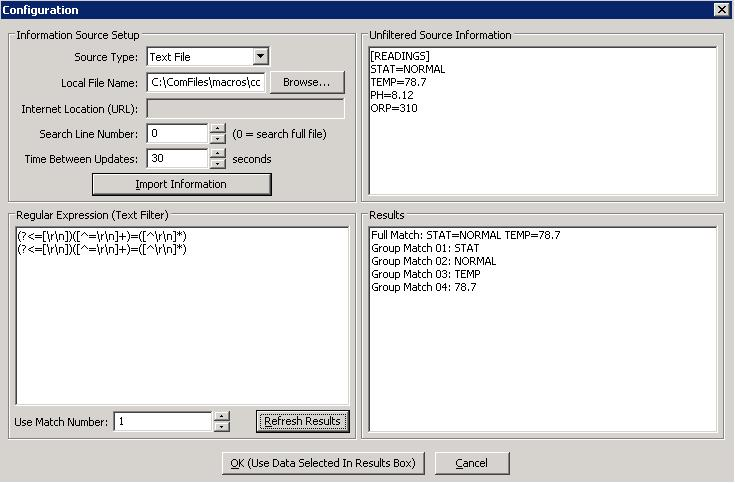# Pulling data from txt/ini file - howto on section

#### wiszmaster

##### New member
Guys,

I'm trying to pull some data out of a text file.

the following is what my TXT file looks like:
Code:
``````[READINGS]
STAT=NORMAL
TEMP=79.6
PH=8.23
ORP=445
COND=--.-
[PX1000]
PX1_1=79.7
PX2_1=119
PX2_2=---
PX2_3=317
PX2_4=---
PX3_1=6.27``````

I'm using the following Regular Expression (Text Filter)

Code:
``````(?<=[\r\n])([^=\r\n]+)=([^\r\n]*)
(?<=[\r\n])([^=\r\n]+)=([^\r\n]*)
(?<=[\r\n])([^=\r\n]+)=([^\r\n]*)
(?<=[\r\n])([^=\r\n]+)=([^\r\n]*)
(?<=[\r\n])([^=\r\n]+)=([^\r\n]*)``````I can only go 5 lines deep, until it tells me No Match Found. - it doesn't make it past the
Code:
`` [PX1000]``
.... any idea how i can get past it & read from the 2nd section of the file?

Thank You,

marco
Looking for additional LCD resources? Check out our LCD blog for the latest developments in LCD technology.

#### wiszmaster

##### New member
ok, someone else figured it out - we user the following code:
Code:
``(?<=[\r\n])([^=\r\n]+)=([^\r\n]*)``
and just increased the "Match Number" to get to the lines/variables we need.

Thanks,

marco